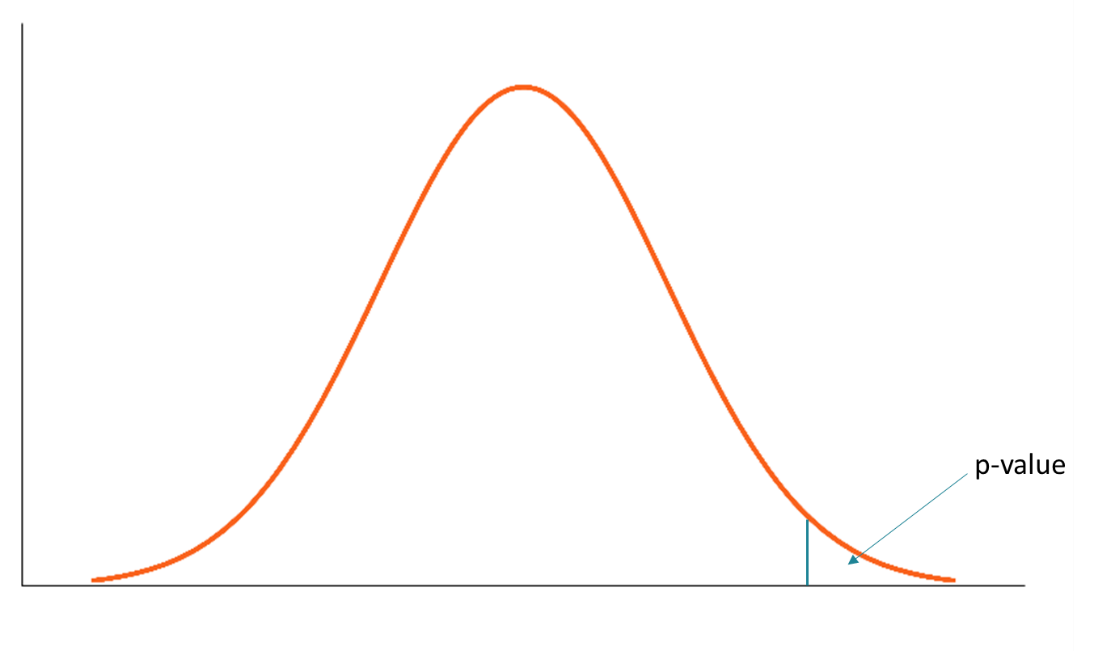## ML context for the day – 18th August 2021

P-Value## Simple linear regression

y = mx + c

y – Dependent variable(DV)

x – Independent variable(IV)

c – constant

## AI Context for the day – 6th August 2021

Colab## Training set and Test set

Training set : A set which is used to train a model.

Test set : A set which is a subset of training set and is used to test the trained model.

Microsoft

Amazon

## Filling missing data using scikit

from sklearn.impute import SimpleImputer
We are importing the simple imputer from scikit library.
imputer = SimpleImputer(missing_values=np.nan, strategy=’mean’)
The strategy used is mean, where the missing data is replaced by average of the values.
imputer.fit(X[:, 1:3])
The number of columns on which the above function is used is 2 i.e between 1 and 3.
X[:, 1:3] = imputer.transform(X[:, 1:3])
The new column is again assigned to the 2 columns.